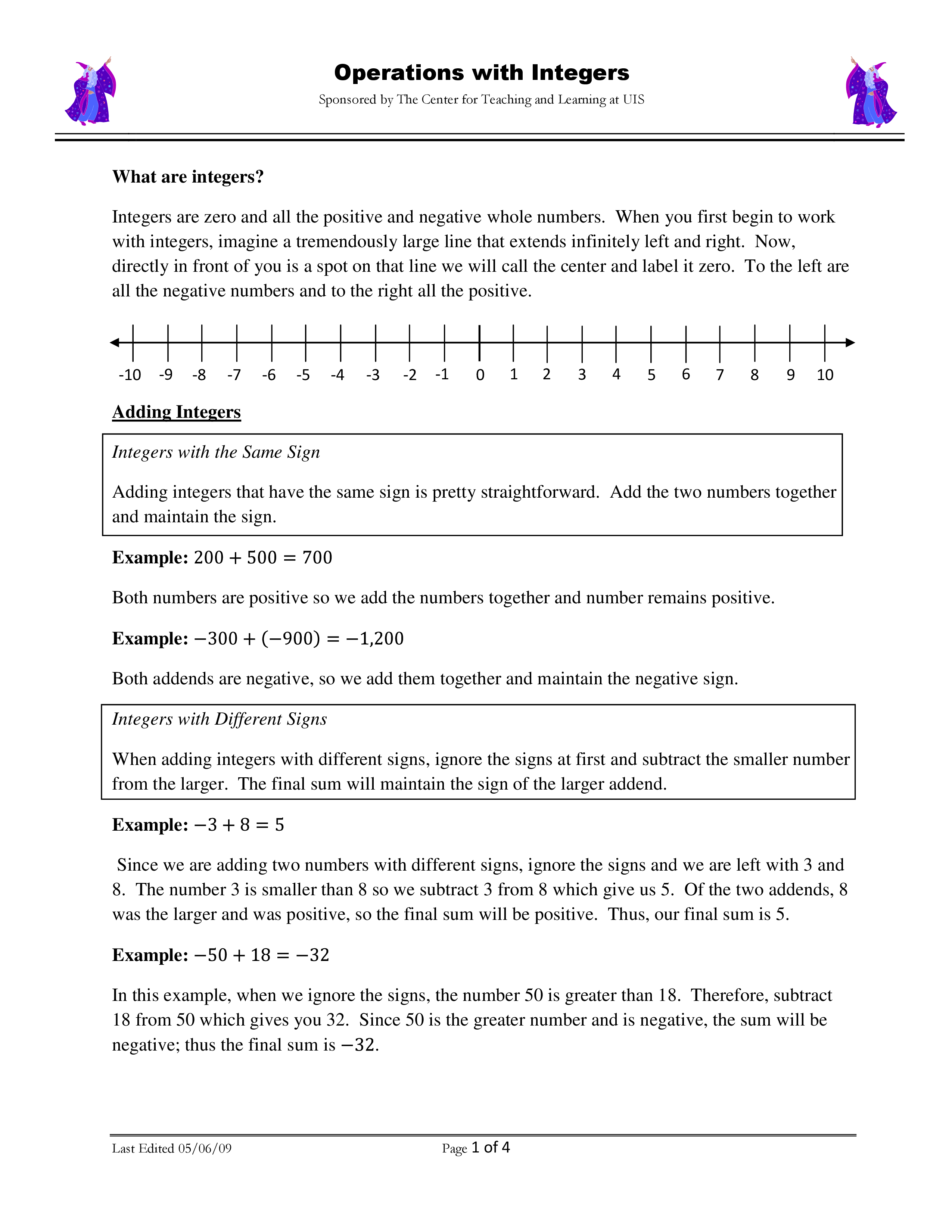Operations with Integers Summary copy 2/27/2019
starstarstarstarstarstarstarstarstarstar
by Matthew Heckmann
| 28 Questions1
1 pt
Absolute value is always positive because it is a distance.
True
False
2
1 pt
The opposite of -2 is
3
1 pt
7 > -4
True
False
4
1 pt
0 < -5
True
False
5
5 pts
|-12| =
6
5 pts
|-5 + 7| =
7
5 pts
|-6| + |4| =8
9
8
1 pt
9
1 pt
How do you determine the sign when adding a positive and a negative number?10
10
1 pt
Subtract:11
12
11
1 pt
Fill in the blank: When you have an ______ number of negative signs your answer is negative.
12
1 pt
Fill in the blank: When you have an _____ number of negative signs your answer is positive.
13
5 pts
-2 + (-3) =
14
1 pt
True
False
15
1 pt
-9 + 3 =
16
1 pt
A will always be positive
B will always be negative
C will depend on which number is bigger
17
5 pts
6 - (-12)
18
1 pt
6 - (-12) is the same as 6 + 12.
True
False
19
5 pts
2 - 9 =
20
1 pt
2 - 9 is the same as 2 + 9.
True
False
21
5 pts
-2 x -10
22
5 pts
3 x -6
23
1 pt
When you multiply a negative and a positive number, the answer
A will always be positive
B will always be negative
C will depend on which number is bigger
24
5 pts
3(-2)(5) =
25
5 pts
-560 / 7 =
26
1 pt
When you divide two negative numbers, the answer
A will always be positive
B will always be negative
C will depend on which number is bigger
27
1 pt
The sign of the answer to the following problem will be (-6)(4)(-3) =
A positive
B negative
28
1 pt
When multiplying or dividing: If you have an even number of negative signs, the answer will always be positive.
True
False